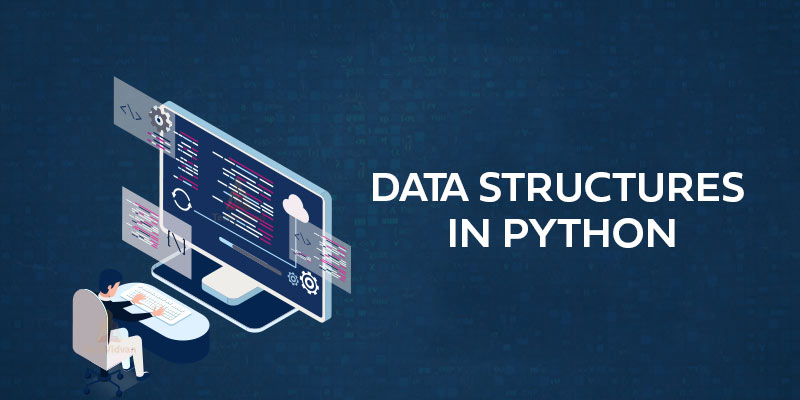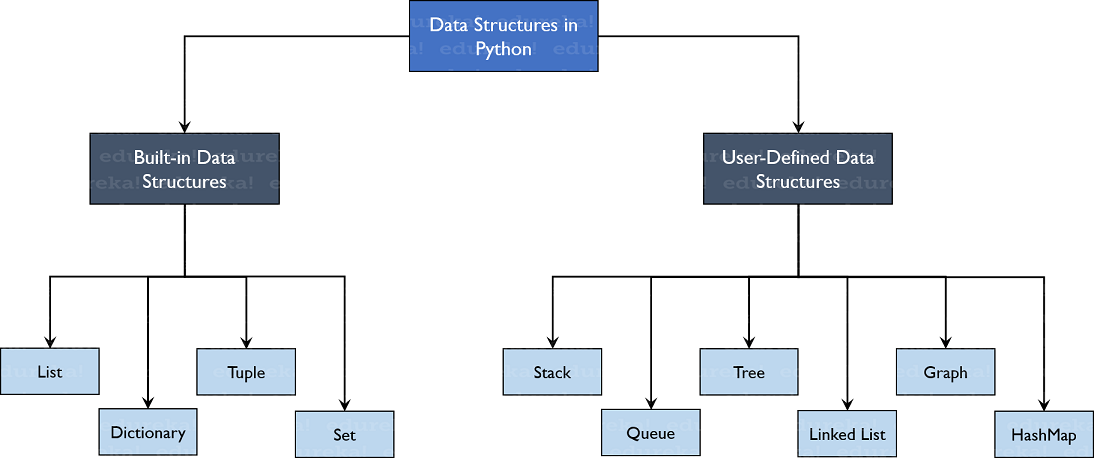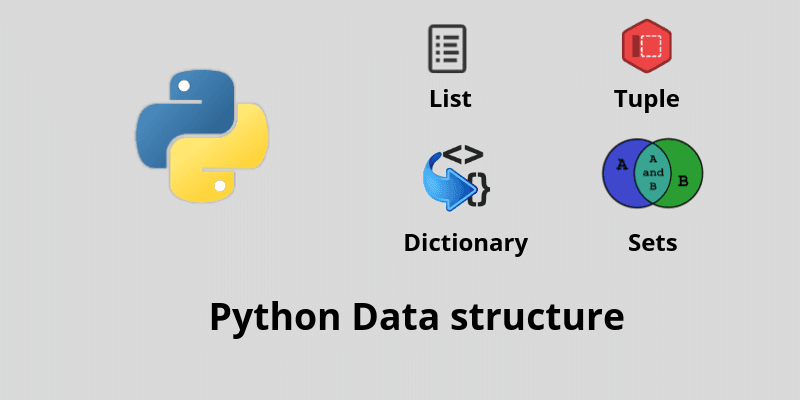This article was published as a part of the Data Science Blogathon

## Introduction

Python Programming Language has been used worldwide for different fields such as making websites, Software EngineeringArtificial Intelligence, Data Science, and much more. But to make all of these things possible, data plays a very important role which means that this data should be stored efficiently and access to it must be timely. So, to achieve this, we use something called Data Structures. So, In this article, we will go through the topics in Data Structures in Python.Image Source:

The topics which we are going to cover in this detailed article are as follows:

• What is meant by Data Structure?
• Why do we need Data Structures?
• What are the Types of Data Structures in Python?
• What are Built-in Data Structures in Python?
• Deep Understanding of Built-in Data Structures in Python

So, Let’s Get Started,

## What is meant by Data Structure?

To enable easier access and efficient modifications, the utmost thing is to Organizing, managing, and storing data. And Data Structures allows us to organize the data in such a way that enables us to store collections of data, relate them and perform operations on them accordingly.

So, with the help of Data Structures, we can organize our data in such a way that it can be retrieved quickly. But one Data Structure is not sufficient to handle all the use case scenarios. That is why we have multiple data structures which can be used for various use cases.

## Why do we need Data Structures?

Consider the following scenario, where you want to search for a particular document in file explorer with over thousands of documents stored. One way to do that is by going one by one in a linear way and find the required document, but this is a time-consuming process.

Another way is to jump directly go to that place where it is stored or where the related documents are present.

YES, your Operating System (OS) does this, using Indexing and hashtables which is a type of data structure. This reduces the time required to search even if there are lots of files present. This is why Data Structures are important.

## What are the Types of Data Structures in Python?

In Python, we have implicit support for Data Structures that enable you to store and access data. In this, the data structures involved are as follows:

• List,
• Tuple,
• Dictionary, and
• Set.

It’s not all but Python also allows its users to create their own Data Structures enabling them to have full control over their functionality. The most prominent Data Structures are

• Stack,
• Queue,
• Tree,
• Graph,
• Hash Map, etc.

All these data structures mentioned above are also available to you in other programming languages such as C, Java, C++, etc. In this article, we have restricted our discussion only up to Built-In Data Structures in Python.All about Different types of Data Structures present in Python

Image Source:

## Built-In Data Structures in Python

As the name suggests, the Data Structures that come under this category are built-in with Python which makes programming easier and helps programmers and Data Scientists to use them so that they can obtain the solutions much faster. The built-in data structures in Python are as follows:

• Lists
• Tuples
• Dictionaries
• SetsImage Source:

## Lists

Lists are the basic data structures and are used to store data of different data types in a sequential manner. During list formation, the Interpreter assigned the addresses to every element of the list called an Index. The index value in the list starts from 0 and goes on until the last element, this index is called the positive index. In Lists, we have also negative indexing which starts from -1 that enables us to access elements from the last to the first. Let us now understand lists and their functionalities in a better way with the help of the following examples.

### Creating a List

To create a list, we can use the square brackets and add elements to it accordingly. If we do not pass any elements inside the square brackets, then we get an empty list as the output.

### For Example –

```# Creating an empty List
empty_list = []
print(empty_list)
# Create a List with some data elements
my_list = ['Analytics Vidhya', 2, 56, 78.453, 8.743]
print(my_list)```

Output:

```[]
['Analytics Vidhya', 2, 56, 78.453, 8.743]```

### Accessing the elements of a List

Accessing the elements of a list is the same as that of accessing the characters of Strings in Python. We have to pass the index values and can obtain the values as needed.

To know more about the string functions in Python, you can refer to my article .

### For Example –

```my_list = ['Analytics Vidhya', 2, 78.453, 'c']
# Accessing the elements one by one using a for loop
for element in my_list:
print(element)
# Access all the elements of a list
print("All elements:", my_list)
# Accessing the index 3 element from the list
print("Index 3 element:", my_list)
# Accessing the first two elements of the list
print("First Two elements:", my_list[0:2])
# Accessing the elements in reverse order
print("All elements in reverse order:", my_list[::-1])```

Output:

```Analytics Vidhya
2
78.453
c
All elements: ['Analytics Vidhya', 2, 78.453, 'c']
Index 3 element: c
First Two elements: ['Analytics Vidhya', 2]
All elements in reverse order: ['c', 78.453, 2, 'Analytics Vidhya']```

### Adding new elements to a List

To add the elements to the list, we can use the append(), and extend() functions.

• The append() function adds all the elements passed to it as a single element.
• The extend() function adds the elements one by one into the list.

### For Example –

```my_list = ['Analytics Vidhya', 2, 78.453, 'c']
# Adding a another list as a single element
my_list.append(['Data Analyst', 'Data Scientist'])
print(my_list)
# Adding the another list elements as different elements
my_list.extend(['Data Analyst', 'Data Scientist'])
print(my_list)```

Output:

```['Analytics Vidhya', 2, 78.453, 'c', ['Data Analyst', 'Data Scientist']]
['Analytics Vidhya', 2, 78.453, 'c', ['Data Analyst', 'Data Scientist'], 'Data Analyst', 'Data Scientist']```

### Adding new elements to a List at a specific Index

To add the new elements at a specific index, we can use the insert() function that adds the element passed to the index value and increases the size of the list too.

### For Example –

```my_list = ['Analytics Vidhya', 2, 78.453, 'c']
# Adding a new list at the second position
my_list.insert(2, ['Data Analyst', 'Data Scientist'])
print(my_list)```

Output:

`['Analytics Vidhya', 2, ['Data Analyst', 'Data Scientist'], 78.453, 'c']`

### Removing the elements from the list at specific Index

To delete the elements at a specific index, we can use the del keyword which is built-in into Python but this does not return anything to us.

### For Example –

```my_list = ['Analytics Vidhya', 2, 78.453, 'c']
# Remove the third element from the list
del my_list
print(my_list)```

Output:

`['Analytics Vidhya', 2, 'c']`

If we also want the element back after removing it from the list, we have to use the pop() function which takes the index value as its arguments.

### For Example –

my_list = [‘Analytics Vidhya’, 2, 78.453, ‘c’]
# Remove the third element from the list
popped_element = my_list.pop(2)
print(my_list)
print(“popped_element:”, popped_element)

Output:

```['Analytics Vidhya', 2, 'c']
popped_element: 78.453```

### Removing the specific elements from the List

To remove an element by its value, we can use the remove() function.

### For Example –

```my_list = ['Analytics Vidhya', 2, 78.453, 'c']
# Remove the 'c' element from the list
my_list.remove('c')
print(my_list)```

Output:

`['Analytics Vidhya', 2, 78.453]`

### Removing all the elements of the List

To remove all the elements of a list, we can use the clear() function, which gives us an empty list after apply on a particular list.

For Example –

```my_list = ['Analytics Vidhya', 2, 78.453, 'c']
# Remove all the elements from the list and make it empty
my_list.clear()
print(my_list)```

Output:

`[]`

### Sorting the List

To sort the list, we use the sort() function. But while sorting you have to confirm that the data types of all the elements are the same since we cannot compare two different data types but you can use a mix of float and int data types while sorting a list.

### For Example –

```my_list = [23,65,76.87,0,23.87]
# Sort all the elements of a list in ascending order
my_list.sort()
print(my_list)```

Output:

`[0, 23, 23.87, 65, 76.87]`

## Tuples

Tuples are somehow the same as that of lists but with the exception that the data once entered into the tuple cannot be changed no matter what i.e, Immutable. The only exception is when we have the data inside the tuple which is mutable (i.e, have a list, etc), only then we can change the tuple data. Let us now understand tuples and their functionalities in a better way with the help of the following examples.

### Creating a Tuple

We can create a tuple using parenthesis or using the tuple() function.

### For Example –

```# Creating an empty tuple
empty_tuple = ()
print(empty_tuple)
print(type(empty_tuple))
# Creating a tuple with some data elements
my_tuple =('Data Scientist', 'Data Analyst', 'ML Engineer', 5)
print(my_tuple)```

Output:

```()

('Data Scientist', 'Data Analyst', 'ML Engineer', 5)```

### Accessing the elements of a Tuple

Accessing the elements of a tuple is the same as it is for accessing elements in lists.

### For Example –

```my_tuple =(1, 56.87, 'Data Scientist', 'Data Analyst', 'ML Engineer', 'c')
# Accessing all the elements of a tuple
for element in my_tuple:
print(element)
# Accessing the first element of a tuple
print("First element:", my_tuple)
# Accessing the last element of a tuple
print("Last element:", my_tuple[-1])
# Accessing the first three elements of a tuple
print("First three elements:", my_tuple[0:3])
# Accessing the characters of a string in a tuple
print(my_tuple)```

Output:

```1
56.87
Data Scientist
Data Analyst
ML Engineer
c
First element: 1
Last element: c
First three elements: (1, 56.87, 'Data Scientist')
A```

### Returns the index of the specified element in a Tuple

To find the index of a particular element in a tuple, we can use the index() function which takes the value of the element as an input for which we have to find the index. If we give an element that is not present in the tuple to index() function, then it gives the ValueError.

### For Example –

```my_tuple =('Data Scientist', 'Data Analyst', 'ML Engineer', '5', 78.64)
print(my_tuple)
print(my_tuple.index('5'))```

Output:

```('Data Scientist', 'Data Analyst', 'ML Engineer', '5', 78.64)
3```

### Returns the occurrence of the element in a tuple

To find how many times a particular element is present in the tuple, we can use the count() function which takes the value of the element as an input for which we have to find the frequency. If we give an element that is not present in the tuple to count() function, then it doesn’t give the error but gives the count of that element to zero.

### For Example –

```my_tuple =(5, 'Data Scientist', 'Data Analyst', 'ML Engineer', '5', 78.64, 5)
print(my_tuple)
print(my_tuple.count(5))```

Output:

```(5, 'Data Scientist', 'Data Analyst', 'ML Engineer', '5', 78.64, 5)
2```

### Adding the new elements to a tuple

To append the values in an existing tuple, we can use the ‘+’ operator, taking another tuple to be appended to it.

### For Example –

```my_tuple =(5, 'Data Scientist', 'Data Analyst', 'ML Engineer', '5', 78.64, 5)
# Adding a single element to a tuple
my_tuple = my_tuple + ('Analytics Vidhya',)
print(my_tuple)
# Adding more than one elements to a tuple
my_tuple = my_tuple + ('Analytics Vidhya','Data Mastermind')
my_tuple```

Output:

```(5, 'Data Scientist', 'Data Analyst', 'ML Engineer', '5', 78.64, 5, 'Analytics Vidhya')
(5,  'Data Scientist',  'Data Analyst',  'ML Engineer',  '5',  78.64,  5,  'Analytics Vidhya',  'Analytics Vidhya',  'Data Mastermind')```

## Dictionaries

Dictionaries are used to store the data in the form of key-value pairs.

### An analogy of a dictionary in real-life

To understand dictionary data structure you can think of a phone directory where we have hundreds and thousands of names of different persons and their corresponding phone numbers have been added. Now here are the constant values (i.e, Name) and the Phone Numbers which we referred to as the keys. And the various names and phone numbers are the values that have been fed to the keys. If we want to access the values of the keys, then we will obtain all the names and phone numbers.

So that is exactly what a key-value pair is. And in Python, this structure is stored using Dictionaries. Let us now understand dictionaries and their functionalities in a better way with the help of the following examples.

### Creating a Dictionary

Dictionaries can be created using the flower braces or using the dict() function. We need to add the key-value pairs whenever we work with dictionaries.

### For Example –

```# Creating an empty Dictionary
empty_dict = {}
print(empty_dict)
# Creating a Dictionary with some data elements
my_dict = {1: 'Analytics Vidhya', 2: 'Data Scientist', 3: 'Data Engineer'}
print(my_dict)```

Output:

```{}
{1: 'Analytics Vidhya', 2: 'Data Scientist', 3: 'Data Engineer'}```

### Returns the value of a Particular Key in a dictionary

We can access the elements of a dictionary using the keys only. We can use either the get() function or just pass the key values and we will be retrieving the values.

### For Example –

```my_dict = {1: 'Analytics Vidhya', 2: 'Data Scientist', 3: 'Data Engineer'}
# Get the value corresponding to key 3```
print(my_dict.get(3))
my_dict

Output:

```Data Engineer
'Data Engineer'```

### Changing and Adding key, value pairs

To change the values of the dictionary, we can take the help of the keys. So, we have to firstly access the key and then change the value accordingly. To add values, we simply just add another key-value pair (See the example shown below).

### For Example –

```my_dict = {'First': 'Analytics', 'Second': 'Machine Learning', 'Third': 'Data Science'}
print(my_dict)
# Changing the element
my_dict['Second'] = 'Deep Learning'
print(my_dict)
my_dict['Third'] = 'Data Mastermind'
print(my_dict)```

Output:

```{'First': 'Analytics', 'Second': 'Machine Learning', 'Third': 'Data Science'}
{'First': 'Analytics', 'Second': 'Deep Learning', 'Third': 'Data Science'}
{'First': 'Analytics', 'Second': 'Deep Learning', 'Third': 'Data Mastermind'}```

### Returns the set of elements of a dictionary

To find the set of elements in a dictionary, we can use the items() function, which gives all the key-value pairs of a dictionary.

### For Example –

```my_dict = {1: 'Analytics Vidhya', 2: 'Data Scientist', 3: 'Data Engineer'}
print(my_dict.items())```

Output:

`dict_items([(1, 'Analytics Vidhya'), (2, 'Data Scientist'), (3, 'Data Engineer')])`

### Returns all the keys present in a dictionary

To find all the keys present in a dictionary, we can use the keys() function, which gives all the keys of a dictionary.

### For Example –

```my_dict = {1: 'Analytics Vidhya', 2: 'Data Scientist', 3: 'Data Engineer'}
print(my_dict.keys())```

Output:

`dict_keys([1, 2, 3])`

### Returns all the values of the keys present in a dictionary

To find all the values present in a dictionary, we can use the values() function, which gives all the values of a dictionary.

### For Example –

```my_dict = {1: 'Analytics Vidhya', 2: 'Data Scientist', 3: 'Data Engineer'}
print(my_dict.values())```

Output:

`dict_values(['Analytics Vidhya', 'Data Scientist', 'Data Engineer'])`

### Deleting Key-Value pairs in a dictionary

Deleting Values: To delete the values, we can use the pop() function which returns the value that has been deleted.

Deleting Key-Value pair: To retrieve the key-value pair, we can use the popitem() function which returns a tuple key and value.

Deleting the Entire Dictionary: To clear the entire dictionary, we can use the clear() function.

### For Example –

```my_dict = {'First': 'Analytics', 'Second': 'Machine Learning', 'Third': 'Data Science'}
# Pop the specified element
a = my_dict.pop('Third')
print('Value:', a)
print('Dictionary:', my_dict)
# Pop the complete key-value pair
b = my_dict.popitem()
print('Key, value pair:', b)
print('Dictionary', my_dict)
# Make the dictionary Empty
my_dict.clear()
print('Empty Dictionary', my_dict)```

Output:

```Value: Data Science
Dictionary: {'First': 'Analytics', 'Second': 'Machine Learning'}
Key, value pair: ('Second', 'Machine Learning')
Dictionary {'First': 'Analytics'}
Empty Dictionary {}```

## Sets

To know all about the operations and functionalities involved in Python Sets, you can refer to the following article link, which I published a couple of days back in Analytics Vidhya. I have included a detailed discussion of Python Sets in this article. So, please refer to this article completely and learn all the data structures in Python and accelerate your programming career.

## Other Blog Posts by Me

You can also check my previous blog posts.

Previous Data Science Blog posts.

Here is my Linkedin profile in case you want to connect with me. I’ll be happy to be connected with you.

## Email

For any queries, you can mail me on Gmail.

## End Notes